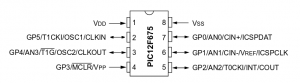# Pulse Width Modulation

MicroControllers are digital devices and usually do not have built in digital to analog converters (or DACs) to output an analog signal. Some, however, do have analog input. For digital output, the voltage is one of two values. Output voltage is either 0 volts or near equal to the voltage source (‘off’ or ‘on’). Using a technique called pulse width modulation (or PWM), a microcontroller can create a ‘simulated’ analog output voltage.

Pulse width modulation turns the output on and off, each with set times at high frequencies to achieve an analog voltage. If you are unfamiliar with pulse width modulation, there are many tutorials on the internet. (Click HERE to search Google for PWM. An excellent one for the Arduino is HERE.)

Here is a short video of pulse width modulation on a PIC12F675 captured on an MSO-19 oscilloscope:

As can be seen in the video, the duty cycle (ratio of ‘on’ versus ‘off’)  goes back and forth from 100% to 0%.

Here is the MPLAB XC8 C source code for creating that PWM signal on a PIC12F675:

The original code was generated with my PIC Timer0 Code Generator and Calculator for a PIC12F675:and then modified to vary the duty cycle. An MSO-19 oscilloscope was used to capture the  PWM signal. If LEDs were placed on the pins of the PIC12F675, they would be seen gradually going from bright to dim and back again to bright.

Using pulse width modulation, an output voltage can be produced anywhere between zero volts and the source voltage. Its simply a matter of math!  For example, if  the source voltage is 5 volts,  to create a 2.5 volt output,  the output pin would be ‘on’ half of the time and ‘off’ half of the time.   (5  ÷ 2 = 2.5)  Of course the frequency of the switching cycle has to be significant.